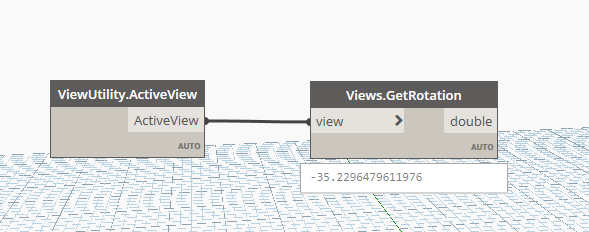# Get view rotation in python like Views.GetRotation

Hey,

I want to get the rotation of a view using python.
DynaMEP has a Node for this called “Views.GetRotation”.Hi @MelleH,

The `View` object has a `UpDirection` property that returns a XYZ object, that in turns has the AngleTo method to get the angle between itself and another XYZ vector.

so it should be something like

``````from pyrevit.revit import doc, DB

angle = doc.ActiveView.UpDirection.AngleTo(DB.XYZ(0, 1, 0))
``````
3 Likes

Thanks. This worked!

Hey,

So this code kinda works but not the way i want it toAs shown below i have 3 sopeboxes i apply a view to.
The center scopebox has 0 degree rotation. which the code correctly reads.
The lef and right scopeboxes both have a 30 degree rotation but in different directions.
The code reads both of the views as 30 degrees.
I expect one of them to be -30 or 330 degrees.

Hello @MelleH ,

Try this:

``````from pyrevit.revit import doc, DB

# Get the two vectors
vectorA = doc.ActiveView.UpDirection
vectorB = DB.XYZ(0, 1, 0)

# Calculate the angle in radians

# Calculate the cross product
cross_product = vectorA.CrossProduct(vectorB)

# Determine the orientation based on the Z component of the cross product
# and adjust the angle's sign accordingly
if cross_product.Z < 0:

# Convert the angle from radians to degrees using the conversion factor directly